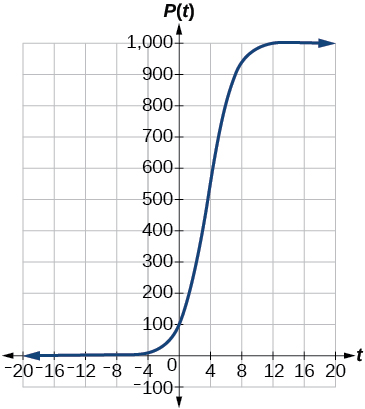6.7 Exponential and logarithmic models  (Page 9/16)

 Page 9 / 16

Graph the function.What is the initial population of fish?

To the nearest tenth, what is the doubling time for the fish population?

about $\text{\hspace{0.17em}}1.4\text{\hspace{0.17em}}$ years

To the nearest whole number, what will the fish population be after $\text{\hspace{0.17em}}2\text{\hspace{0.17em}}$ years?

To the nearest tenth, how long will it take for the population to reach $\text{\hspace{0.17em}}900?$

about $\text{\hspace{0.17em}}7.3\text{\hspace{0.17em}}$ years

What is the carrying capacity for the fish population? Justify your answer using the graph of $\text{\hspace{0.17em}}P.$

Extensions

A substance has a half-life of 2.045 minutes. If the initial amount of the substance was 132.8 grams, how many half-lives will have passed before the substance decays to 8.3 grams? What is the total time of decay?

$4\text{\hspace{0.17em}}$ half-lives; $\text{\hspace{0.17em}}8.18\text{\hspace{0.17em}}$ minutes

The formula for an increasing population is given by $\text{\hspace{0.17em}}P\left(t\right)={P}_{0}{e}^{rt}\text{\hspace{0.17em}}$ where $\text{\hspace{0.17em}}{P}_{0}\text{\hspace{0.17em}}$ is the initial population and $\text{\hspace{0.17em}}r>0.\text{\hspace{0.17em}}$ Derive a general formula for the time t it takes for the population to increase by a factor of M .

Recall the formula for calculating the magnitude of an earthquake, $\text{\hspace{0.17em}}M=\frac{2}{3}\mathrm{log}\left(\frac{S}{{S}_{0}}\right).$ Show each step for solving this equation algebraically for the seismic moment $\text{\hspace{0.17em}}S.$

What is the y -intercept of the logistic growth model $\text{\hspace{0.17em}}y=\frac{c}{1+a{e}^{-rx}}?\text{\hspace{0.17em}}$ Show the steps for calculation. What does this point tell us about the population?

Prove that $\text{\hspace{0.17em}}{b}^{x}={e}^{x\mathrm{ln}\left(b\right)}\text{\hspace{0.17em}}$ for positive $\text{\hspace{0.17em}}b\ne 1.$

Let $\text{\hspace{0.17em}}y={b}^{x}\text{\hspace{0.17em}}$ for some non-negative real number $\text{\hspace{0.17em}}b\text{\hspace{0.17em}}$ such that $\text{\hspace{0.17em}}b\ne 1.\text{\hspace{0.17em}}$ Then,

Real-world applications

For the following exercises, use this scenario: A doctor prescribes 125 milligrams of a therapeutic drug that decays by about 30% each hour.

To the nearest hour, what is the half-life of the drug?

Write an exponential model representing the amount of the drug remaining in the patient’s system after $\text{\hspace{0.17em}}t\text{\hspace{0.17em}}$ hours. Then use the formula to find the amount of the drug that would remain in the patient’s system after 3 hours. Round to the nearest milligram.

$A=125{e}^{\left(-0.3567t\right)};A\approx 43\text{\hspace{0.17em}}$ mg

Using the model found in the previous exercise, find $\text{\hspace{0.17em}}f\left(10\right)\text{\hspace{0.17em}}$ and interpret the result. Round to the nearest hundredth.

For the following exercises, use this scenario: A tumor is injected with $\text{\hspace{0.17em}}0.5\text{\hspace{0.17em}}$ grams of Iodine-125, which has a decay rate of $\text{\hspace{0.17em}}1.15%\text{\hspace{0.17em}}$ per day.

To the nearest day, how long will it take for half of the Iodine-125 to decay?

about $\text{\hspace{0.17em}}60\text{\hspace{0.17em}}$ days

Write an exponential model representing the amount of Iodine-125 remaining in the tumor after $\text{\hspace{0.17em}}t\text{\hspace{0.17em}}$ days. Then use the formula to find the amount of Iodine-125 that would remain in the tumor after 60 days. Round to the nearest tenth of a gram.

A scientist begins with $\text{\hspace{0.17em}}\text{250}\text{\hspace{0.17em}}$ grams of a radioactive substance. After $\text{\hspace{0.17em}}\text{250}\text{\hspace{0.17em}}$ minutes, the sample has decayed to $\text{\hspace{0.17em}}\text{32}\text{\hspace{0.17em}}$ grams. Rounding to five significant digits, write an exponential equation representing this situation. To the nearest minute, what is the half-life of this substance?

$f\left(t\right)=250{e}^{\left(-0.00914t\right)};\text{\hspace{0.17em}}$ half-life: about $\text{\hspace{0.17em}}\text{76}\text{\hspace{0.17em}}$ minutes

The half-life of Radium-226 is $\text{\hspace{0.17em}}1590\text{\hspace{0.17em}}$ years. What is the annual decay rate? Express the decimal result to four significant digits and the percentage to two significant digits.

The half-life of Erbium-165 is $\text{\hspace{0.17em}}\text{10}\text{.4}\text{\hspace{0.17em}}$ hours. What is the hourly decay rate? Express the decimal result to four significant digits and the percentage to two significant digits.

$r\approx -0.0667,$ So the hourly decay rate is about $\text{\hspace{0.17em}}6.67%$

A laser rangefinder is locked on a comet approaching Earth. The distance g(x), in kilometers, of the comet after x days, for x in the interval 0 to 30 days, is given by g(x)=250,000csc(π30x). Graph g(x) on the interval [0, 35]. Evaluate g(5)  and interpret the information. What is the minimum distance between the comet and Earth? When does this occur? To which constant in the equation does this correspond? Find and discuss the meaning of any vertical asymptotes.
The sequence is {1,-1,1-1.....} has
how can we solve this problem
Sin(A+B) = sinBcosA+cosBsinA
Prove it
Eseka
Eseka
hi
Joel
June needs 45 gallons of punch. 2 different coolers. Bigger cooler is 5 times as large as smaller cooler. How many gallons in each cooler?
7.5 and 37.5
Nando
find the sum of 28th term of the AP 3+10+17+---------
I think you should say "28 terms" instead of "28th term"
Vedant
the 28th term is 175
Nando
192
Kenneth
if sequence sn is a such that sn>0 for all n and lim sn=0than prove that lim (s1 s2............ sn) ke hole power n =n
write down the polynomial function with root 1/3,2,-3 with solution
if A and B are subspaces of V prove that (A+B)/B=A/(A-B)
write down the value of each of the following in surd form a)cos(-65°) b)sin(-180°)c)tan(225°)d)tan(135°)
Prove that (sinA/1-cosA - 1-cosA/sinA) (cosA/1-sinA - 1-sinA/cosA) = 4
what is the answer to dividing negative index
In a triangle ABC prove that. (b+c)cosA+(c+a)cosB+(a+b)cisC=a+b+c.
give me the waec 2019 questionsByByByBy